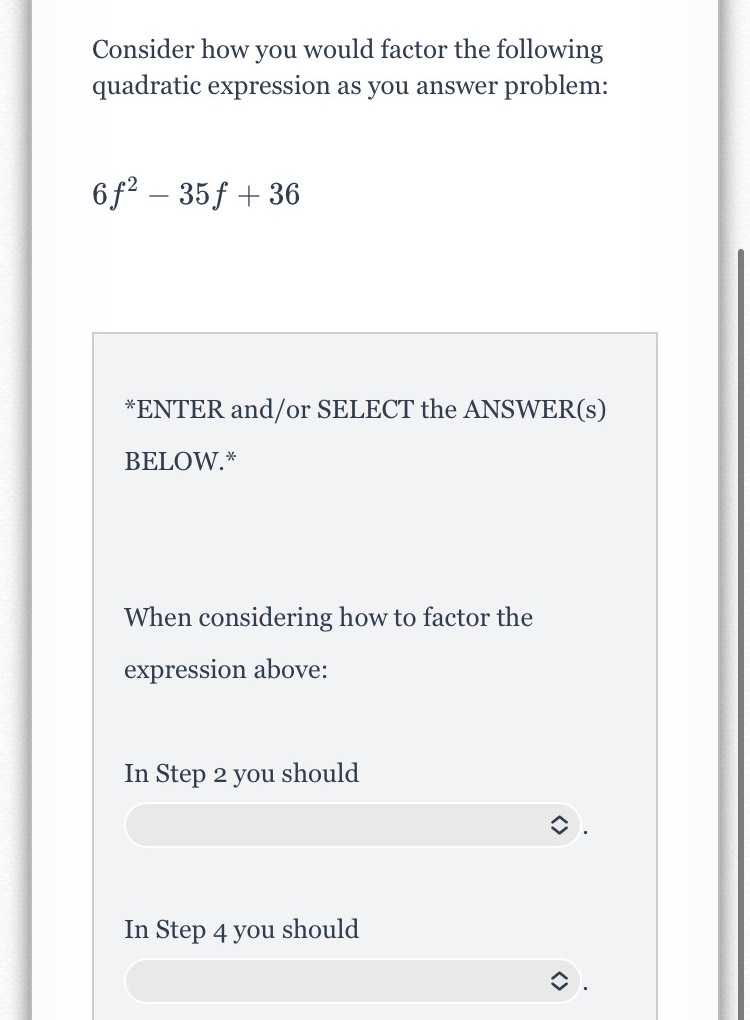### Still have math questions?

Algebra
QuestionConsider how you would factor the following quadratic expression as you answer problem:

$$6 f ^ { 2 } - 35 f + 36$$

When considering how to factor the expression above: I

n Step $$2$$ you should

In Step $$4$$ you should

$$\left(2f- 9\right)\times \left(3f- 4\right)$$